# Pythagorean Theorem

• Introduction and theorem

• Solved Problems: applying the Pythagoras' Theorem

## Introduction

Pythagoras' Theorem: Given a right triangle with sides a and b and a hypotenuse h (the side opposite the right angle). Then,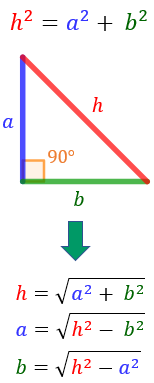Remember that:

• A right triangle (right-angled triangle in British English) is a triangle with a right angle (that is, an angle whose measure is $$\frac{\pi}{2}$$ rad - 90º).

• The hypotenuse is the opposite side as the right angle.

Note: h is always bigger than the other sides (i.e., h > a and h > b).

The Pythagorean theorem is one of the most known results in mathematics and also one of the oldest known. For instance, the pyramid of Kefrén (XXVI century b. C) was built on the base of the so called sacred Egyptian triangle, a right angled triangle of sides 3,4 and 5. The meaning of the theorem can be easily understood, and there are hundreds of proofs of this theorem. As we will see in the exercises of this section, there are numerous applications of the theorem in real life. In addition, the theorem has uses in advanced mathematics as well (vectorial analysis, functional analysis…).

# Solved Problems

Exercise 1

Calculate the hypotenuse of the triangle with sides of 3cm and 4 cm.

Show solution

Exercise 2

If the hypotenuse of a triangle measures 2 cm and one of its sides measures 1cm, what does the other side measure?

Show solution

Exercise 3

Find the measure of the hypotenuse of a right angled triangle whose sides measure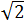and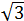.

Show solution

Exercise 4

Find the height of the following triangle whose sides areand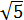, and whose base is 3.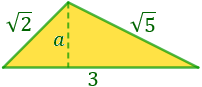Show solution

Exercise 5

Calculate the perimeter of the rhombus if we know its diagonals (height and width) measure 16 and 12 cm, respectively.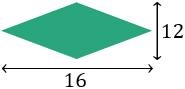Show solution

Exercise 6

Calculate the height that we can reach with a 3m ladder leaning against a wall if the bottom of the ladder is 70cm from the wall.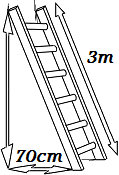Show solution

Exercise 7

The distance Sun-Earth-Moon: Let us suppose that The Moon is in the first quarter phase, which means that from Earth we see it the following way:The white side of The Moon is the side we see (the side illuminated by The Sun).

We know that the distance from Earth to The Moon is 384100Km, and the Earth to The Sun is around 150 million Kilometers. We wish to calculate the distance between The Moon and The Sun in this phase (considering the distances from the centers). Lay out the exercise, but it is not necessary to calculate a result.

Show solution